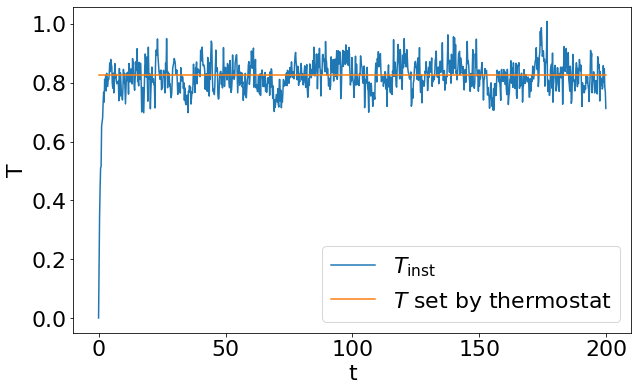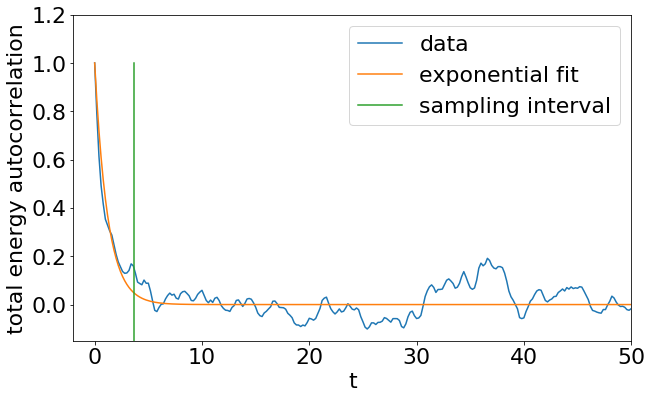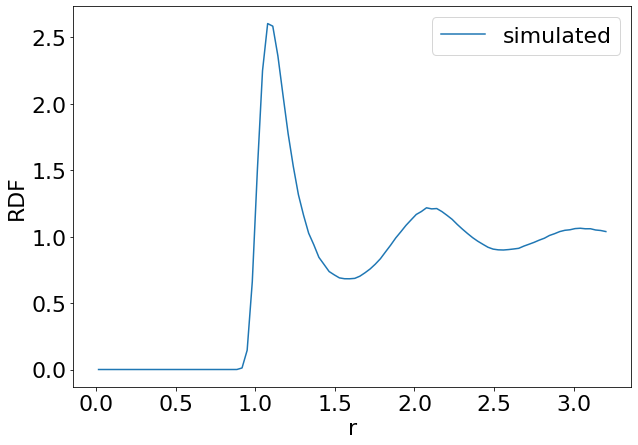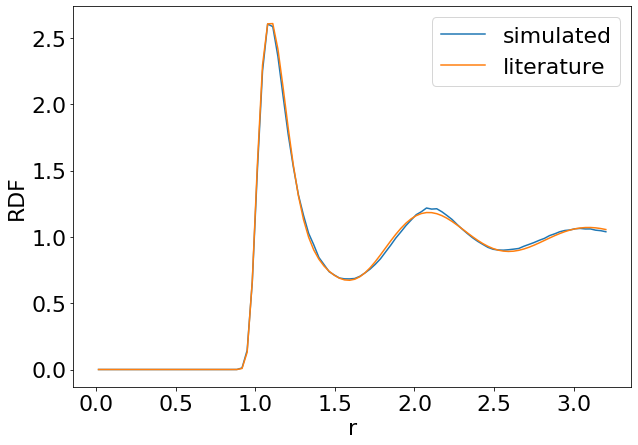# Tutorial 1: Lennard-Jones Liquid¶

## Introduction¶

Welcome to the basic ESPResSo tutorial!

In this tutorial, you will learn, how to use the ESPResSo package for your research. We will cover the basics of ESPResSo, i.e., how to set up and modify a physical system, how to run a simulation, and how to load, save and analyze the produced simulation data.

More advanced features and algorithms available in the ESPResSo package are described in additional tutorials.

## Background¶

Today's research on Soft Condensed Matter has brought the needs for having a flexible, extensible, reliable, and efficient (parallel) molecular simulation package. For this reason ESPResSo (Extensible Simulation Package for Research on Soft Matter Systems)  has been developed at the Max Planck Institute for Polymer Research, Mainz, and at the Institute for Computational Physics at the University of Stuttgart in the group of Prof. Dr. Christian Holm [2,3]. The ESPResSo package is probably the most flexible and extensible simulation package in the market. It is specifically developed for coarse-grained molecular dynamics (MD) simulation of polyelectrolytes but is not necessarily limited to this. For example, it could also be used to simulate granular media. ESPResSo has been nominated for the Heinz-Billing-Preis for Scientific Computing in 2003 .

## The Lennard-Jones Potential¶

A pair of neutral atoms or molecules is subject to two distinct forces in the limit of large separation and small separation: an attractive force at long ranges (van der Waals force, or dispersion force) and a repulsive force at short ranges (the result of overlapping electron orbitals, referred to as Pauli repulsion from the Pauli exclusion principle). The Lennard-Jones potential (also referred to as the L-J potential, 6-12 potential or, less commonly, 12-6 potential) is a simple mathematical model that represents this behavior. It was proposed in 1924 by John Lennard-Jones. The L-J potential is of the form

\begin{equation} V(r) = 4\epsilon \left[ \left( \dfrac{\sigma}{r} \right)^{12} - \left( \dfrac{\sigma}{r} \right)^{6} \right] \end{equation}

where $\epsilon$ is the depth of the potential well and $\sigma$ is the (finite) distance at which the inter-particle potential is zero and $r$ is the distance between the particles. The $\left(\frac{1}{r}\right)^{12}$ term describes repulsion and the $(\frac{1}{r})^{6}$ term describes attraction. The Lennard-Jones potential is an approximation. The form of the repulsion term has no theoretical justification; the repulsion force should depend exponentially on the distance, but the repulsion term of the L-J formula is more convenient due to the ease and efficiency of computing $r^{12}$ as the square of $r^6$.

In practice, the L-J potential is typically cutoff beyond a specified distance $r_{c}$ and the potential at the cutoff distance is zero.

## Units¶

Novice users must understand that ESPResSo has no fixed unit system. The unit system is set by the user. Conventionally, reduced units are employed, in other words LJ units.

## First steps¶

What is ESPResSo? It is an extensible, efficient Molecular Dynamics package specially powerful on simulating charged systems. In depth information about the package can be found in the relevant sources [1,4,2,3].

ESPResSo consists of two components. The simulation engine is written in C++ for the sake of computational efficiency. The steering or control level is interfaced to the kernel via an interpreter of the Python scripting languages.

The kernel performs all computationally demanding tasks. Before all, integration of Newton's equations of motion, including calculation of energies and forces. It also takes care of internal organization of data, storing the data about particles, communication between different processors or cells of the cell-system.

The scripting interface (Python) is used to setup the system (particles, boundary conditions, interactions etc.), control the simulation, run analysis, and store and load results. The user has at hand the full reliability and functionality of the scripting language. For instance, it is possible to use the SciPy package for analysis and PyPlot for plotting. With a certain overhead in efficiency, it can also be bused to reject/accept new configurations in combined MD/MC schemes. In principle, any parameter which is accessible from the scripting level can be changed at any moment of runtime. In this way methods like thermodynamic integration become readily accessible.

Note: This tutorial assumes that you already have a working ESPResSo installation on your system. If this is not the case, please consult the first chapters of the user's guide for installation instructions.

## Overview of a simulation script¶

Typically, a simulation script consists of the following parts:

• System setup (box geometry, thermodynamic ensemble, integrator parameters)
• Placing the particles
• Setup of interactions between particles
• Warm up (bringing the system into a state suitable for measurements)
• Integration loop (propagate the system in time and record measurements)

### System setup¶

The functionality of ESPResSo for python is provided via a python module called espressomd. At the beginning of the simulation script, it has to be imported.

In :
import espressomd
required_features = ["LENNARD_JONES"]
espressomd.assert_features(required_features)
from espressomd import observables, accumulators, analyze

In :
# Importing other relevant python modules
import numpy as np
import matplotlib.pyplot as plt

In :
from scipy import optimize
np.random.seed(42)
plt.rcParams.update({'font.size': 22})

In :
# System parameters
N_PART = 200
DENSITY = 0.75

BOX_L = np.power(N_PART / DENSITY, 1.0 / 3.0) * np.ones(3)


The next step would be to create an instance of the System class. This instance is used as a handle to the simulation system. At any time, only one instance of the System class can exist.

Exercise:

• Create an instance of an espresso system and store it in a variable called system; use BOX_L as box length.
In :
system = espressomd.System(box_l=BOX_L)

In :
# Test solution of Exercise 1
assert isinstance(system, espressomd.System)


It can be used to store and manipulate the crucial system parameters like the time step and the size of the simulation box (time_step, and box_l).

In :
SKIN = 0.4
TIME_STEP = 0.01

system.time_step = TIME_STEP
system.cell_system.skin = SKIN


### Placing and accessing particles¶

Particles in the simulation can be added and accessed via the part property of the System class. Individual particles are referred to by an integer id, e.g., system.part. If id is unspecified, an unused particle id is automatically assigned. It is also possible to use common python iterators and slicing operations to add or access several particles at once.

Particles can be grouped into several types, so that, e.g., a binary fluid can be simulated. Particle types are identified by integer ids, which are set via the particles' type attribute. If it is not specified, zero is implied.

• Create N_PART particles at random positions.

Either write a loop or use an (N_PART x 3) array for positions. Use np.random.random() to generate random numbers.

In :
system.part.add(type= * N_PART, pos=np.random.random((N_PART, 3)) * system.box_l)

Out:
<espressomd.particle_data.ParticleSlice at 0x7fd5f96f3640>
In :
# Test that now we have indeed N_PART particles in the system
assert len(system.part) == N_PART


The particle properties can be accessed using standard numpy slicing syntax:

In :
# Access position of a single particle
print("position of particle with id 0:", system.part.pos)

# Iterate over the first five particles for the purpose of demonstration.
# For accessing all particles, use a slice: system.part[:]
for i in range(5):
print("id", i, "position:", system.part[i].pos)
print("id", i, "velocity:", system.part[i].v)

# Obtain all particle positions
cur_pos = system.part[:].pos

position of particle with id 0: [2.41076339 6.1193638  4.7115492 ]
id 0 position: [2.41076339 6.1193638  4.7115492 ]
id 0 velocity: [0. 0. 0.]
id 1 position: [3.85332274 1.00422894 1.00407369]
id 1 velocity: [0. 0. 0.]
id 2 position: [0.37386074 5.57522583 3.86913442]
id 2 velocity: [0. 0. 0.]
id 3 position: [4.55757705 0.13249407 6.24291778]
id 3 velocity: [0. 0. 0.]
id 4 position: [5.35809689 1.36674105 1.17033384]
id 4 velocity: [0. 0. 0.]


Many objects in ESPResSo have a string representation, and thus can be displayed via python's print function:

In :
print(system.part)

ParticleHandle([('id', 0), ('pos', (2.4107633923737293, 6.119363804209853, 4.711549202763617)), ('_id', 0), ('bonds', ()), ('dip', (0.0, 0.0, 0.0)), ('dipm', 0.0), ('director', (0.0, 0.0, 1.0)), ('exclusions', ()), ('ext_force', (0.0, 0.0, 0.0)), ('ext_torque', (0.0, 0.0, 0.0)), ('f', (0.0, 0.0, 0.0)), ('fix', (0, 0, 0)), ('gamma', (-1.0, -1.0, -1.0)), ('gamma_rot', (-1.0, -1.0, -1.0)), ('image_box', (0, 0, 0)), ('mass', 1.0), ('mol_id', 0), ('mu_E', (0.0, 0.0, 0.0)), ('node', 0), ('omega_body', (0.0, 0.0, 0.0)), ('omega_lab', (0.0, 0.0, 0.0)), ('q', 0.0), ('quat', (1.0, 0.0, 0.0, 0.0)), ('rinertia', (1.0, 1.0, 1.0)), ('rotation', (0, 0, 0)), ('swimming', {'v_swim': 0.0, 'f_swim': 0.0, 'mode': 'N/A', 'dipole_length': 0.0}), ('temp', -1.0), ('torque_lab', (0.0, 0.0, 0.0)), ('type', 0), ('v', (0.0, 0.0, 0.0)), ('virtual', False), ('vs_quat', array([0., 0., 0., 0.])), ('vs_relative', (0, 0.0, array([0., 0., 0., 0.])))])


### Setting up non-bonded interactions¶

Non-bonded interactions act between all particles of a given combination of particle types. In this tutorial, we use the Lennard-Jones non-bonded interaction. First we define the LJ parameters

In :
# use LJ units: EPS=SIG=1
LJ_EPS = 1.0
LJ_SIG = 1.0
LJ_CUT = 2.5 * LJ_SIG


In a periodic system it is in general not straight forward to calculate all non-bonded interactions. Due to the periodicity and to speed up calculations usually a cut-off $r_{cut}$ for infinite-range potentials like Lennard-Jones is applied, such that $V(r>r_c) = 0$. The potential can be shifted to zero at the cutoff value to ensure continuity using the shift='auto' option of espressomd.interactions.LennardJonesInteraction. To allow for comparison with the fundamental work on MD simulations of LJ systems  we don't shift the potential to zero at the cutoff and instead correct for the long-range error $V_\mathrm{lr}$ later.

To avoid spurious self-interactions of particles with their periodic images one usually forces that the shortest box length is at least twice the cutoff distance:

In :
assert (BOX_L - 2 * SKIN > LJ_CUT).all()


Exercise:

• Setup a Lennard-Jones interaction with $\epsilon=$LJ_EPS and $\sigma=$LJ_SIG that is cut at $r_\mathrm{c}=$LJ_CUT$\times\sigma$ and not shifted.

Hint:

• Have a look at the docs
In :
system.non_bonded_inter[0, 0].lennard_jones.set_params(
epsilon=LJ_EPS, sigma=LJ_SIG, cutoff=LJ_CUT, shift=0)


### Energy minimization¶

In many cases, including this tutorial, particles are initially placed randomly in the simulation box. It is therefore possible that particles overlap, resulting in a huge repulsive force between them. In this case, integrating the equations of motion would not be numerically stable. Hence, it is necessary to remove this overlap. This is typically done by performing a steepest descent minimization of the potential energy until a maximal force criterion is reached.

Note: Making sure a system is well equilibrated highly depends on the system's details. In most cases a relative convergence criterion on the forces and/or energies works well but you might have to make sure that the total force is smaller than a threshold value f_max at the end of the minimization. Depending on the simulated system other strategies like simulations with small time step or capped forces might be necessary.

In :
F_TOL = 1e-2
DAMPING = 30
MAX_STEPS = 10000
MAX_DISPLACEMENT = 0.01 * LJ_SIG
EM_STEP = 10


Exercise:

• Use espressomd.integrate.set_steepest_descent to relax the initial configuration. Use a maximal displacement of MAX_DISPLACEMENT. A damping constant gamma = DAMPING usually is a good choice.
• Use the relative change of the systems maximal force as a convergence criterion. See the documentation espressomd.particle_data module on how to obtain the forces. The steepest descent has converged if the relative force change < F_TOL
• Break the minimization loop after a maximal number of MAX_STEPS steps or if convergence is achieved. Check for convergence every EMSTEP steps.

Hint: To obtain the initial forces one has to initialize the integrator using integ_steps=0, i.e. call system.integrator.run(0) before the force array can be accessed.

In :
# Set up steepest descent integration
system.integrator.set_steepest_descent(f_max=0,  # use a relative convergence criterion only
gamma=DAMPING,
max_displacement=MAX_DISPLACEMENT)

# Initialize integrator to obtain initial forces
system.integrator.run(0)
old_force = np.max(np.linalg.norm(system.part[:].f, axis=1))

while system.time / system.time_step < MAX_STEPS:
system.integrator.run(EM_STEP)
force = np.max(np.linalg.norm(system.part[:].f, axis=1))
rel_force = np.abs((force - old_force) / old_force)
print(f'rel. force change:{rel_force:.2e}')
if rel_force < F_TOL:
break
old_force = force

rel. force change:1.00e+00
rel. force change:9.99e-01
rel. force change:9.38e-01
rel. force change:6.00e-01
rel. force change:6.81e-01
rel. force change:5.53e-01
rel. force change:3.49e-01
rel. force change:2.62e-01
rel. force change:2.26e-03

In :
# check that after the exercise the total energy is negative
assert system.analysis.energy()['total'] < 0
# reset clock
system.time = 0.


### Choosing the thermodynamic ensemble, thermostat¶

Simulations can be carried out in different thermodynamic ensembles such as NVE (particle Number, Volume, Energy), NVT (particle Number, Volume, Temperature) or NpT-isotropic (particle Number, pressure, Temperature).

In this tutorial, we use the Langevin thermostat.

In :
# Parameters for the Langevin thermostat
# reduced temperature T* = k_B T / LJ_EPS
TEMPERATURE = 0.827  # value from Tab. 1 in 
GAMMA = 1.0


Exercise:

• Use system.integrator.set_vv() to use a Velocity Verlet integration scheme and system.thermostat.set_langevin() to turn on the Langevin thermostat.

Set the temperature to TEMPERATURE and damping coefficient to GAMMA.

For details see the online documentation.

In :
system.integrator.set_vv()
system.thermostat.set_langevin(kT=TEMPERATURE, gamma=GAMMA, seed=42)


### Integrating equations of motion and taking manual measurements¶

Now, we integrate the equations of motion and take measurements of relevant quantities.

In :
# Integration parameters
STEPS_PER_SAMPLE = 20
N_SAMPLES = 1000

times = np.zeros(N_SAMPLES)
e_total = np.zeros_like(times)
e_kin = np.zeros_like(times)
T_inst = np.zeros_like(times)


Exercise:

• Integrate the system and measure the total and kinetic energy. Take N_SAMPLES measurements every STEPS_PER_SAMPLE integration steps.
• Calculate the total and kinetic energies using the analysis method system.analysis.energy().
• Use the containers times, e_total and e_kin from the cell above to store the time series.
• From the simulation results, calculate the instantaneous temperature $T_{\mathrm{inst}} = 2/3 \times E_\mathrm{kin}$/N_PART.
In :
for i in range(N_SAMPLES):
times[i] = system.time
energy = system.analysis.energy()
e_total[i] = energy['total']
e_kin[i] = energy['kinetic']
system.integrator.run(STEPS_PER_SAMPLE)
T_inst = 2. / 3. * e_kin / N_PART

In :
plt.figure(figsize=(10, 6))
plt.plot(times, T_inst, label='$T_{\\mathrm{inst}}$')
plt.plot(times, [TEMPERATURE] * len(times), label='$T$ set by thermostat')
plt.legend()
plt.xlabel('t')
plt.ylabel('T')
plt.show()Since the ensemble average $\langle E_\text{kin}\rangle=3/2 N k_B T$ is related to the temperature, we may compute the actual temperature of the system via $k_B T= 2/(3N) \langle E_\text{kin}\rangle$. The temperature is fixed and does not fluctuate in the NVT ensemble! The instantaneous temperature is calculated via $2/(3N) E_\text{kin}$ (without ensemble averaging), but it is not the temperature of the system.

In the first simulation run we picked STEPS_PER_SAMPLE arbitrary. To ensure proper statistics at short total run time, we will now calculate the steps_per_uncorrelated_sample which we will use for the rest of the tutorial.

In :
# Use only the data after the equilibration period in the beginning
warmup_time = 15
e_total = e_total[times > warmup_time]
e_kin = e_kin[times > warmup_time]
times = times[times > warmup_time]
times -= times

In :
def autocor(x):
x = np.asarray(x)
mean = x.mean()
var = np.var(x)
xp = x - mean
corr = analyze.autocorrelation(xp) / var
return corr

def fit_correlation_time(data, ts):
data = np.asarray(data)
data /= data

def fitfn(t, t_corr): return np.exp(-t / t_corr)
popt, pcov = optimize.curve_fit(fitfn, ts, data)
return popt


Exercise

• Calculate the autocorrelation of the total energy (store in e_total_autocor). Calculate the correlation time (corr_time) and estimate a number of steps_per_uncorrelated_sample for uncorrelated sampling

Hint

• we consider samples to be uncorrelated if the time between them is larger than 3 times the correlation time
In :
e_total_autocor = autocor(e_total)
corr_time = fit_correlation_time(e_total_autocor[:100], times[:100])
steps_per_uncorrelated_sample = int(np.ceil(3 * corr_time / system.time_step))

In :
print(steps_per_uncorrelated_sample)

363


We plot the autocorrelation function and the fit to visually confirm a roughly exponential decay

In :
plt.figure(figsize=(10, 6))
plt.plot(times, e_total_autocor, label='data')
plt.plot(times, np.exp(-times / corr_time), label='exponential fit')
plt.plot(2 * [steps_per_uncorrelated_sample * system.time_step],
[min(e_total_autocor), 1], label='sampling interval')
plt.xlim(left=-2, right=50)
plt.ylim(top=1.2, bottom=-0.15)
plt.legend()
plt.xlabel('t')
plt.ylabel('total energy autocorrelation')
plt.show()For statistical analysis, we only want uncorrelated samples.

Exercise:

• Calculate the mean and standard error of the mean potential energy per particle from uncorrelated samples (define mean_pot_energy and SEM_pot_energy).

Hint

• you know how many steps are between samples in e_total and how many steps are between uncorrelated samples. So you have to figure out how many samples to skip
In :
uncorrelated_sample_step = int(np.ceil(steps_per_uncorrelated_sample / STEPS_PER_SAMPLE))
pot_energies = (e_total - e_kin)[::uncorrelated_sample_step] / N_PART
mean_pot_energy = np.mean(pot_energies)
SEM_pot_energy = np.std(pot_energies) / np.sqrt(len(pot_energies))

In :
print(f'mean potential energy = {mean_pot_energy:.2f} +- {SEM_pot_energy:.2f}')

mean potential energy = -4.97 +- 0.01


For comparison to literature values we need to account for the error made by the LJ truncation. For an isotropic system one can assume that the density is homogeneous behind the cutoff, which allows to calculate the so-called long-range corrections to the energy and pressure, $$V_\mathrm{lr} = 1/2 \rho \int_{r_\mathrm{c}}^\infty 4 \pi r^2 g(r) V(r) \,\mathrm{d}r,$$ Using that the radial distribution function $g(r)=1$ for $r>r_\mathrm{cut}$ one obtains $$V_\mathrm{lr} = -\frac{8}{3}\pi \rho \varepsilon \sigma^3 \left[\frac{1}{3} (\sigma/r_{cut})^9 - (\sigma/r_{cut})^3 \right].$$ Similarly, a long-range contribution to the pressure can be derived .

In :
tail_energy_per_particle = 8. / 3. * np.pi * DENSITY * LJ_EPS * \
LJ_SIG**3 * (1. / 3. * (LJ_SIG / LJ_CUT)**9 - (LJ_SIG / LJ_CUT)**3)
mean_pot_energy_corrected = mean_pot_energy + tail_energy_per_particle
print(f'corrected mean potential energy = {mean_pot_energy_corrected:.2f}')

corrected mean potential energy = -5.37


This value differs quite strongly from the uncorrected one but agrees well with the literature value $U^i = -5.38$ given in Table 1 of Ref. .

### Automated data collection¶

As we have seen, it is easy to manually extract information from an ESPResSo simulation, but it can get quite tedious. Therefore, ESPResSo provides a number of data collection tools to make life easier (and less error-prone). We will now demonstrate those with the calculation of the radial distribution function.

Observables extract properties from the particles and calculate some quantity with it, e.g. the center of mass, the total energy or a histogram.

Accumulators allow the calculation of observables while running the system and then doing further analysis. Examples are a simple time series or more advanced methods like correlators. For our purposes we need an accumulator that calculates the average of the RDF samples.

In :
# Parameters for the radial distribution function
N_BINS = 100
R_MIN = 0.0
R_MAX = system.box_l / 2.0


Exercise

• Instantiate a RDF observable
• Instantiate a MeanVarianceCalculator accumulator to track the RDF over time. Samples should be taken every steps_per_uncorrelated_sample steps.
• Add the accumulator to the auto_update_accumulators of the system for automatic updates
In :
rdf_obs = observables.RDF(ids1=system.part[:].id, min_r=R_MIN, max_r=R_MAX, n_r_bins=N_BINS)
rdf_acc = accumulators.MeanVarianceCalculator(obs=rdf_obs, delta_N=steps_per_uncorrelated_sample)


Now we don't need an elaborate integration loop anymore, instead the RDFs are calculated and accumulated automatically

In :
system.integrator.run(N_SAMPLES * steps_per_uncorrelated_sample)


Exercise

• Get the mean RDF (define rdf) from the accmulator
• Get the histogram bin centers (define rs) from the observable
In :
rdf = rdf_acc.mean()
rs = rdf_obs.bin_centers()

In :
fig, ax = plt.subplots(figsize=(10, 7))
ax.plot(rs, rdf, label='simulated')
plt.legend()
plt.xlabel('r')
plt.ylabel('RDF')

Out:
Text(0, 0.5, 'RDF')We now plot the experimental radial distribution. Empirical radial distribution functions have been determined for pure fluids , mixtures  and confined fluids . We will compare our distribution $g(r)$ to the theoretical distribution $g(r^*, \rho^*, T^*)$ of a pure fluid .

In :
# comparison to literature
def calc_literature_rdf(rs, temperature, density, LJ_eps, LJ_sig):
T_star = temperature / LJ_eps
rho_star = density * LJ_sig**3

# expression of the factors Pi from Equations 2-8 with coefficients qi from Table 1
# expression for a,g
def P(q1, q2, q3, q4, q5, q6, q7, q8, q9): return \
q1 + q2 * np.exp(-q3 * T_star) + q4 * np.exp(-q5 * T_star) + q6 / rho_star + q7 / rho_star**2 \
+ q8 * np.exp(-q3 * T_star) / rho_star**3 + q9 * \
np.exp(-q5 * T_star) / rho_star**4
a = P(9.24792, -2.64281, 0.133386, -1.35932, 1.25338,
0.45602, -0.326422, 0.045708, -0.0287681)
g = P(0.663161, -0.243089, 1.24749, -2.059, 0.04261,
1.65041, -0.343652, -0.037698, 0.008899)

# expression for c,k
def P(q1, q2, q3, q4, q5, q6, q7, q8): return \
q1 + q2 * np.exp(-q3 * T_star) + q4 * rho_star + q5 * rho_star**2 + q6 * rho_star**3 \
+ q7 * rho_star**4 + q8 * rho_star**5
c = P(-0.0677912, -1.39505, 0.512625, 36.9323, -
36.8061, 21.7353, -7.76671, 1.36342)
k = P(16.4821, -0.300612, 0.0937844, -61.744,
145.285, -168.087, 98.2181, -23.0583)

# expression for b,h
def P(q1, q2, q3): return q1 + q2 * np.exp(-q3 * rho_star)
b = P(-8.33289, 2.1714, 1.00063)
h = P(0.0325039, -1.28792, 2.5487)

# expression for d,l
def P(q1, q2, q3, q4): return q1 + q2 * \
np.exp(-q3 * rho_star) + q4 * rho_star
d = P(-26.1615, 27.4846, 1.68124, 6.74296)
l = P(-6.7293, -59.5002, 10.2466, -0.43596)

# expression for s
def P(q1, q2, q3, q4, q5, q6, q7, q8): return \
(q1 + q2 * rho_star + q3 / T_star + q4 / T_star**2 + q5 / T_star**3) \
/ (q6 + q7 * rho_star + q8 * rho_star**2)
s = P(1.25225, -1.0179, 0.358564, -0.18533,
0.0482119, 1.27592, -1.78785, 0.634741)

# expression for m
def P(q1, q2, q3, q4, q5, q6): return \
q1 + q2 * np.exp(-q3 * T_star) + q4 / T_star + \
q5 * rho_star + q6 * rho_star**2
m = P(-5.668, -3.62671, 0.680654, 0.294481, 0.186395, -0.286954)

# expression for n
def P(q1, q2, q3): return q1 + q2 * np.exp(-q3 * T_star)
n = P(6.01325, 3.84098, 0.60793)

# fitted expression (=theoretical curve)
# slightly more than 1 to smooth out the discontinuity in the range [1.0, 1.02]
theo_rdf_cutoff = 1.02

theo_rdf = 1 + 1 / rs**2 * (np.exp(-(a * rs + b)) * np.sin(c * rs + d)
+ np.exp(-(g * rs + h)) * np.cos(k * rs + l))
theo_rdf[np.nonzero(rs <= theo_rdf_cutoff)] = \
s * np.exp(-(m * rs + n)**4)[np.nonzero(rs <= theo_rdf_cutoff)]
return theo_rdf

In :
theo_rdf = calc_literature_rdf(rs, TEMPERATURE, DENSITY, LJ_EPS, LJ_SIG)

In :
ax.plot(rs, theo_rdf, label='literature')
ax.legend()
fig

Out:## Further Exercises¶

### Binary Lennard-Jones Liquid¶

A two-component Lennard-Jones liquid can be simulated by placing particles of two types (0 and 1) into the system. Depending on the Lennard-Jones parameters, the two components either mix or separate.

1. Modify the code such that half of the particles are of type=1. Type 0 is implied for the remaining particles.
2. Specify Lennard-Jones interactions between type 0 particles with other type 0 particles, type 1 particles with other type 1 particles, and type 0 particles with type 1 particles (set parameters for system.non_bonded_inter[i,j].lennard_jones where {i,j} can be {0,0}, {1,1}, and {0,1}. Use the same Lennard-Jones parameters for interactions within a component, but use a different lj_cut_mixed parameter for the cutoff of the Lennard-Jones interaction between particles of type 0 and particles of type 1. Set this parameter to $2^{\frac{1}{6}}\sigma$ to get de-mixing or to $2.5\sigma$ to get mixing between the two components.
3. Record the radial distribution functions separately for particles of type 0 around particles of type 0, type 1 around particles of type 1, and type 0 around particles of type 1. This can be done by changing the ids1/ids2 arguments of the espressomd.observables.RDF command. You can record all three radial distribution functions in a single simulation. It is also possible to write them as several columns into a single file.
4. Plot the radial distribution functions for all three combinations of particle types. The mixed case will differ significantly, depending on your choice of lj_cut_mixed. Explain these differences.

 http://espressomd.org
 HJ Limbach, A. Arnold, and B. Mann. ESPResSo: An extensible simulation package for research on soft matter systems. Computer Physics Communications, 174(9):704–727, 2006.
 A. Arnold, O. Lenz, S. Kesselheim, R. Weeber, F. Fahrenberger, D. Rohm, P. Kosovan, and C. Holm. ESPResSo 3.1 — molecular dynamics software for coarse-grained models. In M. Griebel and M. A. Schweitzer, editors, Meshfree Methods for Partial Differential Equations VI, volume 89 of Lecture Notes in Computational Science and Engineering, pages 1–23. Springer Berlin Heidelberg, 2013.
 A. Arnold, BA Mann, HJ Limbach, and C. Holm. ESPResSo–An Extensible Simulation Package for Research on Soft Matter Systems. Forschung und wissenschaftliches Rechnen, 63:43–59, 2003.
 W. P. Allen & D. J. Tildesley. Computer Simulation of Liquids. Oxford University Press, 2017.
 L. Verlet, “Computer ‘Experiments’ on Classical Fluids. I. Thermodynamical Properties of Lennard-Jones Molecules, Phys. Rev., 159(1):98–103, 1967. DOI:10.1103/PhysRev.159.98
 Morsali, Goharshadi, Mansoori, Abbaspour. An accurate expression for radial distribution function of the Lennard-Jones fluid. Chemical Physics, 310(1–3):11–15, 2005. DOI:10.1016/j.chemphys.2004.09.027
 Matteoli. A simple expression for radial distribution functions of pure fluids and mixtures. The Journal of Chemical Physics, 103(11):4672, 1995. DOI:10.1063/1.470654
 Abbaspour, Akbarzadeha, Abroodia. A new and accurate expression for the radial distribution function of confined Lennard-Jones fluid in carbon nanotubes. RSC Advances, 5(116): 95781–95787, 2015. DOI:10.1039/C5RA16151G